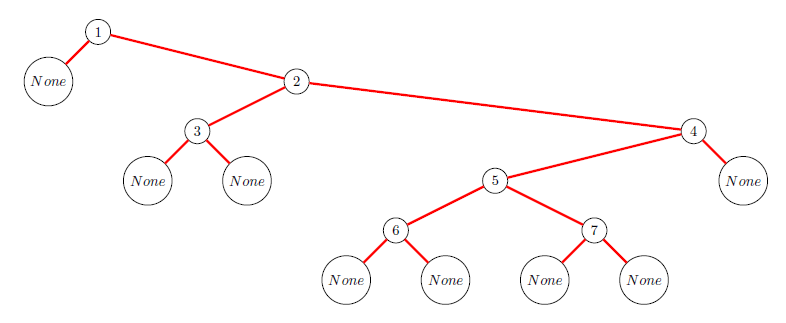# Plotting LabelledOrderedTrees in Knuth style

Consider the tree

t = Permutation([1,3,2,6,5,7,4]).increasing_tree().as_ordered_tree()


A nice simple plot is given by

ascii_art(t)

_________1___________
/                    /
None      ___________2_____________
/                        /
_3___               ______4_______
/    /              /             /
None None       ____5______       None
/          /
_6___      _7___
/    /     /    /
None None  None None


What I need is a graph-plot in exactly this layout in Knuth style (cf. the tables in 7.2.1.6) where the inner nodes (above labeled by numbers) are filled black disks and the leaves (above labeld by 'None') are empty squares.

How can I achieve this?

edit retag close merge delete

There is now also "unicode_art" (not answering the question).

Frédéric, in my jupyter notebook I see no difference although I expected one after looking into abstract_tree.py. What are the requirements?

Ah, I see now, it works with 7.3 (but did not with 7.2). But it does not work with LabelledBinaryTrees. With t = Permutation([1,3,2,6,5,7,4]).increasing_tree() I get the AttributeError 'UnicodeArt' object has no attribute '_root'.

Maybe you could use "t.map_labels(lambda x: 0 if x in ZZ else 1)" to avoid part of the post-processing.

Sort by » oldest newest most voted

This is not an answer but a workaround in the case that one wishes a standalone picture.

Instead of ascii_art(t) execute pretty_print(t). Then edit the TeX code like this:

• Replace commands of the type

\newcommand{\nodec}{\node[draw,circle] (c) {\$None\$} by

\newcommand{\nodeb}{\node[draw,rectangle] (c) {$$} • Replace commands of the type \newcommand{\nodei}{\node[draw,circle] (i) {\6\} by \newcommand{\nodei}{\node[fill=black,draw,circle] (i) {$$}

• Replace

\matrix[column sep=.3cm, row sep=.3cm,ampersand replacement=\&] by

\matrix[row sep=0.7cm,ampersand replacement=\&]

• Replace

\path[ultra thick, red] by

\path[black]

This will replace the tree in Sage styleby a tree in Knuth style:I really would like to see this as the default style for binary trees.

more